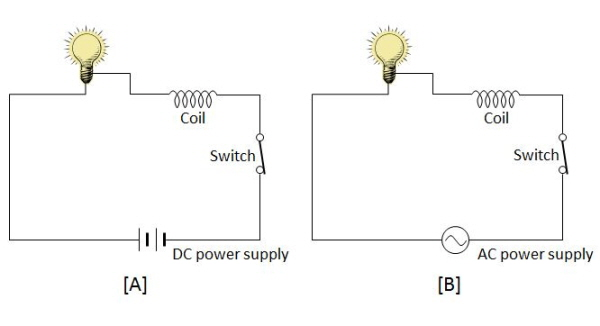# Current CoilThe above shows two circuits with the same light bulb and coil connected to the DC power supply and AC power supply, respectively. The voltage levels of [A] and [B] are the same. Which of the following statements is correct?

a) The intensity of the magnetic field inside the coil of [B] is constant.

b) The intensity of the current flowing in the light bulb of [A] is greater than that of [B].

c) The light bulb of [B] is brighter than that of [A].

×